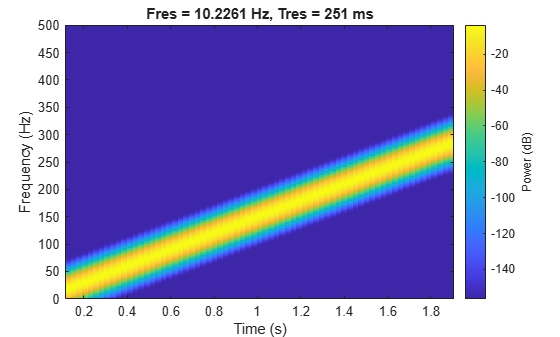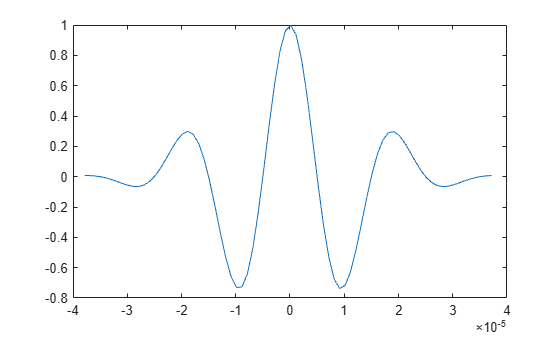# Common Aperiodic Waveforms

Signal Processing Toolbox™ provides functions for generating several widely used aperiodic waveforms.

• `gauspuls` generates a Gaussian-modulated sinusoidal pulse with a specified time, center frequency, and fractional bandwidth. Optional parameters return in-phase and quadrature pulses, the RF signal envelope, and the cutoff time for the trailing pulse envelope.

• `chirp` generates a linear, logarithmic, or quadratic swept-frequency sinusoidal signal. An optional parameter specifies alternative sweep methods. An optional parameter allows an initial phase to be specified in degrees.

Compute 2 seconds of a linear chirp signal with a sample rate of 1 kHz that starts at DC and crosses 150 Hz at 1 second.

```t = 0:1/1000:2; y = chirp(t,0,1,150);```

Plot the spectrogram of the chirp. Specify 90% of overlap between adjoining windowed segments.

`pspectrum(y,t,'spectrogram','OverlapPercent',90)`Use `gauspuls` to plot a 50 kHz Gaussian RF pulse with 60% bandwidth, sampled at a rate of 1 MHz. Truncate the pulse where the envelope falls 40 dB below the peak.

```tc = gauspuls('cutoff',50e3,0.6,[],-40); t = -tc : 1e-6 : tc; yi = gauspuls(t,50e3,0.6); plot(t,yi)```